Search by Topic

Resources tagged with Odd and even numbers similar to Domino Number Patterns:

Filter by: Content type:
Age range:
Challenge level:

There are 64 results

Broad Topics > Numbers and the Number System > Odd and even numbersWhat Number?

Age 5 to 7 Short Challenge Level:

I am less than 25. My ones digit is twice my tens digit. My digits add up to an even number.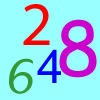Largest Even

Age 5 to 7 Challenge Level:

How would you create the largest possible two-digit even number from the digit I've given you and one of your choice?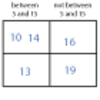More Carroll Diagrams

Age 7 to 11 Challenge Level:

How have the numbers been placed in this Carroll diagram? Which labels would you put on each row and column?Two Numbers Under the Microscope

Age 5 to 7 Challenge Level:

This investigates one particular property of number by looking closely at an example of adding two odd numbers together.Grouping Goodies

Age 5 to 7 Challenge Level:

Pat counts her sweets in different groups and both times she has some left over. How many sweets could she have had?Odd Times Even

Age 5 to 7 Challenge Level:

This problem looks at how one example of your choice can show something about the general structure of multiplication.The Set of Numbers

Age 5 to 7 Challenge Level:

Can you place the numbers from 1 to 10 in the grid?Carroll Diagrams

Age 5 to 11 Challenge Level:

Use the interactivities to fill in these Carroll diagrams. How do you know where to place the numbers?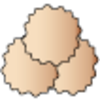Lots of Biscuits!

Age 5 to 7 Challenge Level:

Help share out the biscuits the children have made.One of Thirty-six

Age 5 to 7 Challenge Level:

Can you find the chosen number from the grid using the clues?How Odd

Age 5 to 7 Challenge Level:

This problem challenges you to find out how many odd numbers there are between pairs of numbers. Can you find a pair of numbers that has four odds between them?Pairs of Legs

Age 5 to 7 Challenge Level:

How many legs do each of these creatures have? How many pairs is that?I Like ...

Age 5 to 7 Challenge Level:

Mr Gilderdale is playing a game with his class. What rule might he have chosen? How would you test your idea?Domino Sorting

Age 5 to 7 Challenge Level:

Try grouping the dominoes in the ways described. Are there any left over each time? Can you explain why?Light the Lights

Age 5 to 7 Challenge Level:

Investigate which numbers make these lights come on. What is the smallest number you can find that lights up all the lights?Cube Bricks and Daisy Chains

Age 5 to 7 Challenge Level:

Daisy and Akram were making number patterns. Daisy was using beads that looked like flowers and Akram was using cube bricks. First they were counting in twos.The Thousands Game

Age 7 to 11 Challenge Level:

Each child in Class 3 took four numbers out of the bag. Who had made the highest even number?Multiplication Series: Number Arrays

Age 5 to 11

This article for teachers describes how number arrays can be a useful representation for many number concepts.Number Detective

Age 5 to 11 Challenge Level:

Follow the clues to find the mystery number.Arrangements

Age 7 to 11 Challenge Level:

Is it possible to place 2 counters on the 3 by 3 grid so that there is an even number of counters in every row and every column? How about if you have 3 counters or 4 counters or....?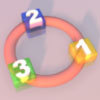More Numbers in the Ring

Age 5 to 7 Challenge Level:

If there are 3 squares in the ring, can you place three different numbers in them so that their differences are odd? Try with different numbers of squares around the ring. What do you notice?Curious Number

Age 7 to 11 Challenge Level:

Can you order the digits from 1-3 to make a number which is divisible by 3 so when the last digit is removed it becomes a 2-figure number divisible by 2, and so on?Domino Pick

Age 5 to 7 Challenge Level:

Are these domino games fair? Can you explain why or why not?Share Bears

Age 5 to 7 Challenge Level:

Yasmin and Zach have some bears to share. Which numbers of bears can they share so that there are none left over?What Do You Need?

Age 7 to 11 Challenge Level:

Four of these clues are needed to find the chosen number on this grid and four are true but do nothing to help in finding the number. Can you sort out the clues and find the number?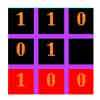Odd Tic Tac

Age 5 to 7 Challenge Level:

An odd version of tic tac toePart the Piles

Age 7 to 11 Challenge Level:

Try to stop your opponent from being able to split the piles of counters into unequal numbers. Can you find a strategy?Always, Sometimes or Never?

Age 5 to 11 Challenge Level:

Are these statements relating to odd and even numbers always true, sometimes true or never true?Venn Diagrams

Age 5 to 11 Challenge Level:

Use the interactivities to complete these Venn diagrams.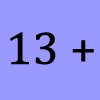What Could it Be?

Age 5 to 7 Challenge Level:

In this calculation, the box represents a missing digit. What could the digit be? What would the solution be in each case?Sets of Four Numbers

Age 7 to 11 Challenge Level:

There are ten children in Becky's group. Can you find a set of numbers for each of them? Are there any other sets?Number Round Up

Age 5 to 7 Challenge Level:

Arrange the numbers 1 to 6 in each set of circles below. The sum of each side of the triangle should equal the number in its centre.Odds and Threes

Age 7 to 11 Challenge Level:

A game for 2 people using a pack of cards Turn over 2 cards and try to make an odd number or a multiple of 3.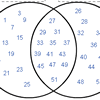Various Venns

Age 7 to 11 Challenge Level:

Use the interactivities to complete these Venn diagrams.Numbers as Shapes

Age 5 to 7 Challenge Level:

Use cubes to continue making the numbers from 7 to 20. Are they sticks, rectangles or squares?Seven Flipped

Age 7 to 11 Challenge Level:

Investigate the smallest number of moves it takes to turn these mats upside-down if you can only turn exactly three at a time.Sorting Numbers

Age 5 to 7 Challenge Level:

Can you sort numbers into sets? Can you give each set a name?Becky's Number Plumber

Age 7 to 11 Challenge Level:

Becky created a number plumber which multiplies by 5 and subtracts 4. What do you notice about the numbers that it produces? Can you explain your findings?Magic Crosses

Age 7 to 14 Challenge Level:

Can you find examples of magic crosses? Can you find all the possibilities?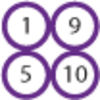Pairs of Numbers

Age 5 to 7 Challenge Level:

If you have ten counters numbered 1 to 10, how many can you put into pairs that add to 10? Which ones do you have to leave out? Why?Play to 37

Age 7 to 11 Challenge Level:

In this game for two players, the idea is to take it in turns to choose 1, 3, 5 or 7. The winner is the first to make the total 37.Square Subtraction

Age 7 to 11 Challenge Level:

Look at what happens when you take a number, square it and subtract your answer. What kind of number do you get? Can you prove it?Ring a Ring of Numbers

Age 5 to 7 Challenge Level:

Choose four of the numbers from 1 to 9 to put in the squares so that the differences between joined squares are odd.Even and Odd

Age 5 to 7 Challenge Level:

This activity is best done with a whole class or in a large group. Can you match the cards? What happens when you add pairs of the numbers together?Number Tracks

Age 7 to 11 Challenge Level:

Ben’s class were cutting up number tracks. First they cut them into twos and added up the numbers on each piece. What patterns could they see?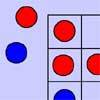Red Even

Age 7 to 11 Challenge Level:

You have 4 red and 5 blue counters. How many ways can they be placed on a 3 by 3 grid so that all the rows columns and diagonals have an even number of red counters?Sets of Numbers

Age 7 to 11 Challenge Level:

How many different sets of numbers with at least four members can you find in the numbers in this box?Down to Nothing

Age 7 to 11 Challenge Level:

A game for 2 or more people. Starting with 100, subratct a number from 1 to 9 from the total. You score for making an odd number, a number ending in 0 or a multiple of 6.A Mixed-up Clock

Age 7 to 11 Challenge Level:

There is a clock-face where the numbers have become all mixed up. Can you find out where all the numbers have got to from these ten statements?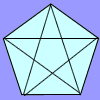Diagonal Trace

Age 7 to 11 Challenge Level:

You can trace over all of the diagonals of a pentagon without lifting your pencil and without going over any more than once. Can the same thing be done with a hexagon or with a heptagon?